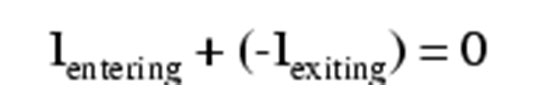## What Is Kirchhoff’s Current Law?

“The algebraic sum of all currents entering and exiting a node must equal zero”.

# Kirchhoff’s Current Law (KCL)

## Chapter 6 - Divider Circuits And Kirchhoff's Laws

### What Is Kirchhoff’s Current Law?

Kirchhoff’s Current Law, often shortened to KCL, states that “The algebraic sum of all currents entering and exiting a node must equal zero.”

This law is used to describe how a charge enters and leaves a wire junction point or node on a wire.

Armed with this information, let’s now take a look at an example of the law in practice, why it’s important, and how it was derived.

### Parallel Circuit Review

Let’s take a closer look at that last parallel example circuit: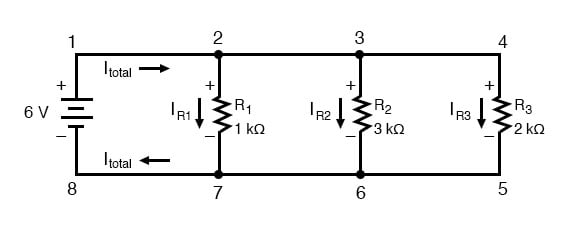Solving for all values of voltage and current in this circuit: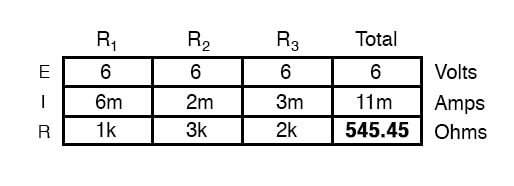At this point, we know the value of each branch current and of the total current in the circuit. We know that the total current in a parallel circuit must equal the sum of the branch currents, but there’s more going on in this circuit than just that. Taking a look at the currents at each wire junction point (node) in the circuit, we should be able to see something else: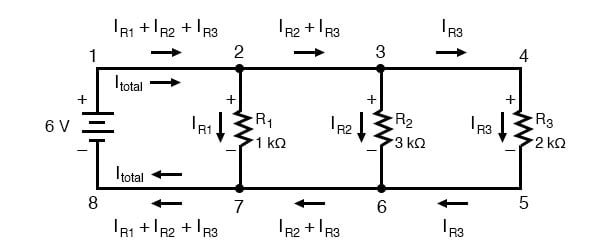### Currents Entering and Exiting a Node

At each node on the positive “rail” (wire 1-2-3-4) we have current splitting off the main flow to each successive branch resistor. At each node on the negative “rail” (wire 8-7-6-5) we have current merging together to form the main flow from each successive branch resistor. This fact should be fairly obvious if you think of the water pipe circuit analogy with every branch node acting as a “tee” fitting, the water flow splitting or merging with the main piping as it travels from the output of the water pump toward the return reservoir or sump.

If we were to take a closer look at one particular “tee” node, such as node 6, we see that the current entering the node is equal in magnitude to the current exiting the node: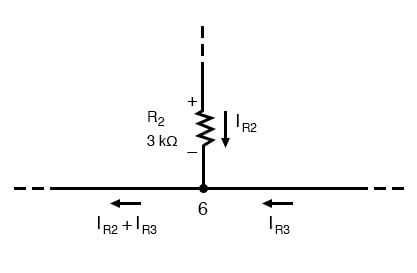From the top and from the right, we have two currents entering the wire connection labeled as node 6. To the left, we have a single current exiting the node equal in magnitude to the sum of the two currents entering. To refer to the plumbing analogy: so long as there are no leaks in the piping, what flow enters the fitting must also exit the fitting. This holds true for any node (“fitting”), no matter how many flows are entering or exiting. Mathematically, we can express this general relationship as such: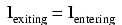### Kirchhoff’s Current Law

Mr. Kirchhoff decided to express it in a slightly different form (though mathematically equivalent), calling it Kirchhoff’s Current Law (KCL):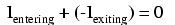Summarized in a phrase, Kirchhoff’s Current Law reads as such:

“The algebraic sum of all currents entering and exiting a node must equal zero”

That is, if we assign a mathematical sign (polarity) to each current, denoting whether they enter (+) or exit (-) a node, we can add them together to arrive at a total of zero, guaranteed.

Taking our example node (number 6), we can determine the magnitude of the current exiting from the left by setting up a KCL equation with that current as the unknown value: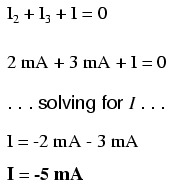The negative (-) sign on the value of 5 milliamps tells us that the current is exiting the node, as opposed to the 2 milliamp and 3 milliamp currents, which must both be positive (and therefore entering the node). Whether negative or positive denotes current entering or exiting is entirely arbitrary, so long as they are opposite signs for opposite directions and we stay consistent in our notation, KCL will work.

Together, Kirchhoff’s Voltage and Current Laws are a formidable pair of tools useful in analyzing electric circuits. Their usefulness will become all the more apparent in a later chapter (“Network Analysis”), but suffice it to say that these Laws deserve to be memorized by the electronics student every bit as much as Ohm’s Law.

REVIEW:

• Kirchhoff’s Current Law (KCL): “The algebraic sum of all currents entering and exiting a node must equal zero”

RELATED WORKSHEETS:

• Share
Published under the terms and conditions of the Design Science License